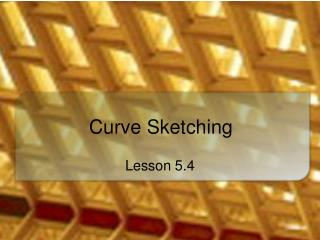DownloadDownload PresentationCurve Sketching

# Curve Sketching

Télécharger la présentation## Curve Sketching

- - - - - - - - - - - - - - - - - - - - - - - - - - - E N D - - - - - - - - - - - - - - - - - - - - - - - - - - -
##### Presentation Transcript

1. Curve Sketching Lesson 5.4

2. Motivation • Graphing calculators decrease the importance of curve sketching So why a lesson on curve sketching? • A calculator graph may be misleading • What happens outside specified window? • Calculator plots, connects points without showing what happens between points • False asymptotes • Curve sketching is a good way to reinforce concepts of lessons in this chapter

3. Tools for Curve Sketching • Test for concavity • Test for increasing/decreasing functions • Critical points • Zeros • Maximums and Minimums

4. Strategy • Determine domain of function • Find y-intercepts, x-intercepts (zeros) • Check for vertical, horizontal asymptotes • Determine values for f '(x) = 0, critical points • Determine f ''(x) • Gives inflection points • Test for intervals of concave up, down • Plot intercepts, critical points, inflection points • Connect points with smooth curve • Check sketch with graphing calculator

5. Using First, Second Derivatives • Note the four possibilities for a function to be … • Increasing or decreasing • Concave up or concave down f '(x) f ''(x)

6. Try It Out • Find as much as you can about the function without graphing it on the calculator

7. Graphing Without the Formula • Consider a function of this description • Can you graph it? • This function is continuous for all reals • A y-intercept at (0, 2)

8. Assignment • Lesson 5.4 • Page 354 • Exercises 1 – 39 odd